# Assignment - Polynomials, Class 10 Mathematics Notes - Class 10

## Class 10: Assignment - Polynomials, Class 10 Mathematics Notes - Class 10

The document Assignment - Polynomials, Class 10 Mathematics Notes - Class 10 is a part of Class 10 category.
All you need of Class 10 at this link: Class 10

1. Look at the graph in fig given below. Each is the graph of y = p (x), where p(x) is a polynomial. For each of the graph, find the numbre of zeros of p(x).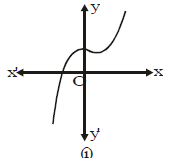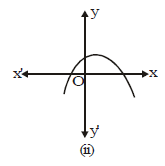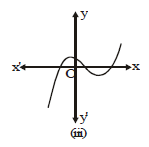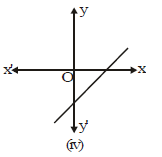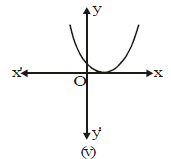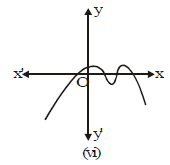2. Consider the cubic polynomial f(x) = x3 – 4x. Find from the fig, the number of zeros of the above stated polynomials.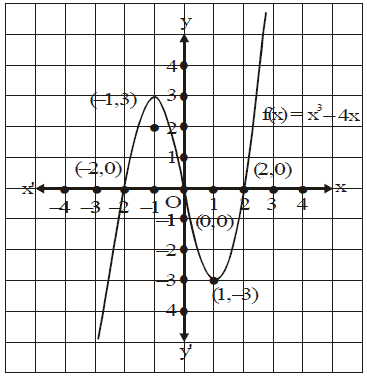3. Let f(x) = x3

The graph of the polynomial is shown in fig.
(i) Find the number of zeros of polynomial f(x).
(ii) Determine the co-ordinates of the points, at which the graph intersects the x-axis.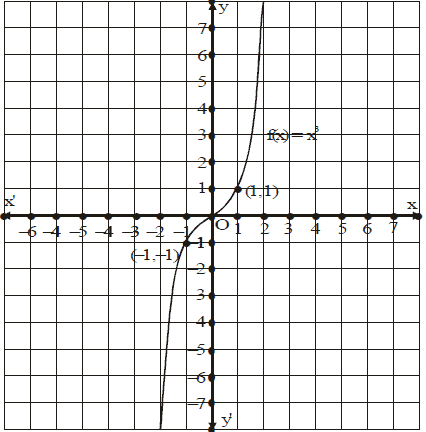1. Find the zeros of the following quadratic polynomials and verify the relationship between the zeros and their coefficients.
(i) 6x2 – x – 1
(ii) 25x (x + 1) + 4
(iii) 4x2 + 4x + 1
(iv) 48y2 – 13y – 1
(v) 63 – 2x – x2
(vi) 2x2 – 5x
(vii)49x2 – 81
(viii) 4x2 – 4x – 3

2. Find a quadratic polynomial each with the given numbers as the zeros of the polynomials.
(i) 3 +√7,3 – √7
(ii) √3,-2√3
(iii) -3/7,-2/3
(iv) √3, 3√3
(v) 2 + 3 √2, 2 – 3 √2
(vi) 8/3 , 5/2

3. Find a quadratic polynomial each with the given numbers as the sum and product of its zeros respectively.
(i) 4√3,9

(ii) 2√3-1,3-√3

(iii) 0,– 1/4

(iv)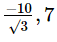(v) 5/6 ,25/9

(vi)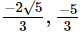(vii)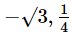(viii) – 6/5 , 9/25
(ix) √2, –12

4. If α and β are the zeros of the polynomial f(x) = 5x2 + 4x – 9 then evaluate the following :

(i) α – β
(ii) α2 + β2
(iii) α2 – β2
(iv) α3 + β3
(v) α3 – β3
(vi) α4 – β4

5. If one of the zeros of the quadratic polynomial 2x2 + px + 4 is 2, find the other zero. Also find the value of p.

6. If one zero of the polynomial (a2 + 9)x2 + 13x + 6a is the reciprocal of the other, find the value of a.

7. If the product of zeros of the polynomial ax2 – 6x – 6 is 4, find the value of a.

8. Find the zeros of the quadratic polynomial 5x2 – 4 – 8x and verify the relationship between the zeros and
the coefficients of the polynomial.
9. Determine if 3 is a zero of p(x) =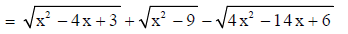10. If α and β be two zeros of the quadratic polynomial ax2 + bx + c, then evaluate :

(i) a2 + b2
(ii) α3 + β3
(iii)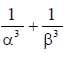(iv)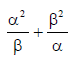11. Find the value of k :
(i) I f α and β are the zeros of the polynomial x2 – 5x + k where α – β = 1.
(ii) If α and β are the zeros of the polynomial x2 – 8x + k such that α2 + β2 = 40.
(iii) If α and β are the zeros of the polynomial x2 – 6x + k such that 3α + 2β = 20.

12. If 2 and 3 are zeros of polynomial 3x2 – 2kx + 2m, find the values of k and m.

13. If one zero of polynomial 3x2 = 8x + 2k + 1 is seven times the other, then find the zeros and the value of k.

14. If α and β are the zeros of the polynomial 2x2 – 4x + 5. Form the polynomial where zeros are :​

(i)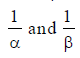(ii)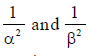(iii)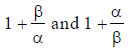15. If α and β are the zeros of the quadratic polynomial x2 – 3x + 2, find a quadratic polynomial whose zeros are :
(i)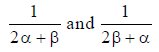(ii)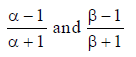16. If the sum of the squares of zeros of the polynomial 5x2 + 3x + k is – 11/25 , find the value of k.

17. If one zero of the quadratic polynomial 2x2 – (3k + 1) x – 9 is negative of the other, find the value of k.

18. If α and β are the two zeros of the quadratic polynomial x2 – 2x + 5, find a quadratic polynomial whose zeros are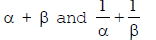19. If α and β are the zeros of the quadratic polynomial f(x) = x2 – px + q, prove that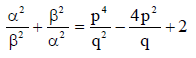20. Apply the division algorithm to find the quotient q(x) and remainder r(x) on dividing p(x) by g(x) as given below :
(i) p(x) = 3x3 + 2x2 + x + 1 ; g(x) = x3 + 3x + 2
(ii) p(x) = x6 + x4 – x2 – 1 ; g(x) = x3 – x2 + x – 1
(iii) p(x) = 2x5 + 3x4 + 4x3 + 4x2 + 3x + 2 ; g(x) = x3 + x2 + x + 1
(iv) p(x) = x3 – 3x2 – x + 3 ; g(x) = x2 – 4x + 3

21. Find the quotient q(x) and remainder r(x) of the following when f(x) is divided by g(x). Verify the division algorithm.
(i) f(x) = x6 + 5x3 + 7x + 3 ; g(x) = x2 + 2
(ii) f(x) = x4 + 2x2 + 1 ; g(x) = x3 + 1
(iii) f(x) = 4x4 – 7x2 + 18x – 1 ; g(x) = 2x + 1
(iv) f(x) = 5x3 – 70x2 + 153x – 342 ; g(x) = x2 – 10x + 6

22. Check whether g(y) is a factor of f(y) by applying the division algorithm :
(i) f(y) = 2y4 + 3y3 – 2y2 – 9y – 12, g(y) = y2 – 3
(ii) f(y) = 3y4 + 5y3 – 7y2 + 2y + 2, g(y) = y2 + 3y + 1
(iii) f(y) = y5 – 4y3 + y2 + 3y + 1, g(y) = y3 – 3y + 1

23. (a) If 1 is the zero of f(x) = k2x2 – 3kx + 3k – 1 then find the value(s) of k.
(b) If 1 and – 2 are the zeros of f(x) = x3 + 10x2 + ax + b, then find the values of a and b.
(c) Find p and q such that 3 and – 1 are the zeros of f(x) = x4 + px3 + qx2 + 12x – 9.
(d) If 3 is the zero of f(x) = x4 – x3 – 8x2 + kx + 12, then find the value of k.
Also show that – 2 is the zero of x3 – 2x + 4

24. (a) Find all the zeros of 3x3 + 16x2 + 23x + 6 if two of its zeros are – 3 and – 2.
(b) Determine all the zeros of 4x3 + 12x2 – x – 3 if two of its zeros are –1/2 and 1/2.
(c) Determine all the zeros of x3 + 5x2 – 2x – 10 if two of its zeros are √2 and  -√2
(d) Determine all the zeros of 4x3 + 12x2 – x – 3 if one of its zeros is 5/2
(e) Determine all the zeros of 4x3 + 5x2 – 180x – 225 if one of its zeros is –5/4 .

25. (a) Find all the zeros of 3x4 – 10x3 + 5x2 + 10x – 8 if three of its zeros are 1, 2 and – 1.
(b) Obtain all the zeros of 2x4 + 5x3 – 8x2 – 17x – 6 if three of its zeros are –1, –3, 2.
(c) Determine all the zeros of x4 – x3 – 8x2 + 2x + 12 if two of its zeros are √2 and -√2.

26. (a) Obtain all other zeros of the polynomial 2x3 – 4x – x2 + 2 if two of its zeros are √2 and – √2 .
(b) Find all the zeros of 2x4 – 9x3 + 5x2 + 3x – 1, if two of its zeros are 2+√3 and 2-√3.
(c) Find all the zeros of the polynomial x4 + x3 – 34x2 – 4x + 120, if two of its zeros are √2 and – √2
(d) Find all the zeros of the polynomial 2x4 + 7x3 – 19x2 – 14x + 30, if two of its zeros are √2 and – √2.

27. (a) On dividing f(x) = 3x3 + x2 + 2x + 5 by a polynomial g(x) = x2 + 2x + 1, the remainder r(x) = 9x + 10.
Find the quotient polynomial q(x).

(b) On dividing f(x) by a polynomial x – 1 – x2, the quotient q(x) and remainder r(x) are (x – 2) and 3 respectively. Find f(x).

(c) On dividing x5 – 4x3 + x2 + 3x + 1 by polynomial g(x), the quotient and remainder are (x2 – 1) and 2 respectively. Find g(x).

(d) On dividing f(x) = 2x5 + 3x4 + 4x3 + 4x2 + 3x + 2 by a polynomial g(x), where g(x) = x3 + x2 + x + 1, the quotient obtained as 2x2 + x + 1. Find the remainder r(x).

PREVIOUS YEARS BOARD (CBSE) QUESTIONS

QUESTIONS CARRYING 1 MARK

1. Write the zeros of the polynomial x2 + 2x + 1.

[Delhi-2008]

2. Write the zeros of the polynomial, x2 – x – 6.

[Delhi-2008]

3. Write a quadratic polynomial, the sum and product of whose zeros are 3 and – 2 respectively.

[Delhi-2008]

4. Write the number of zeros of the polynomial y = f(x) whose graph is given in figure.

[AI-2008]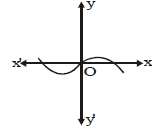5. If (x + a) is a factor of 2x2 + 2ax + 5x + 10, find a [Foreign-2008]

6. For what value of k, (–4) is a zero of the polynomial x2 – x – (2k + 2)?

[Delhi-2009]

7. For what value of p, (–4) is a zero of the polynomial x2 – 2x – (7p + 3)?

[Delhi-2009]

8. If 1 is a zero of the polynomial p(x) = ax2 – 3(a – 1) x – 1, then find the value of a.

[AI-2009]

9. Write the polynomial, the product and sum of whose zeros – 29 and – 23 respectively

[Foreign-2009]

10. Write the polynomial, the product and sum of whose zeros are –153 and – 35 respectively.

[Foreign-2009]

1. (i) One zero, (ii) Two zero, (iii) Three zeros, (iv) One zero, (v) One zero, (vi) Four zeros
2. Three zeros 3. (i) One zero, (ii) (0, 0)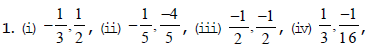(v) 7, –9, (vi) 0, 5/2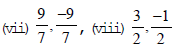2. (i) x2 – 6x + 2 , (ii) x2 – 12, (iii) 21x2 + 23x + 6, (iv) x2 – 4√3x + 9, (v) x2 – 4x – 14, (vi) 6x2 – 31x + 40
3. (i) x2 – 4 √3x + 9, (ii) x2 – 2√3 – 1x + 3 – √3, (iii) 4x2 – 1, (iv) 3x2 + 10√3x + 21, (v) 18x2 – 15x + 50,
(vi) 3x2 + 2 √5x – 5, (vii) 4x2 + 4√3x + 1, (viii) 25x2 + 30x + 9, (ix) x2 – √2x –12

4. (i) 14/5 (ii) 106/25 (iii) -56/25 (iv) -604/125 (v) 854/125 (vi) -5936/125

5. p = – 6, other zero = 1

6. a = 3

7. a = -3/2

8. 2 and -2/5

9. yes

10.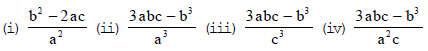11. (i) 6 (ii) 12 (iii) – 16

12. k = 15/2 , m = 9

13.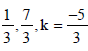14. (i)  1/5 (5x2 – 4x + 2)  (ii) 1/25 (25x2 + 4x + 4)    (iii) 1/5 (5x2 – 8x + 8)

15. (i) 20x2 – 9x + 1 (ii) 3x2 – x

16. 2

17. – 1/3

18. 5x2 – 12x + 4

20. (i) q(x) = 3, r = 2x2 – 8x – 5, (ii) q(x) = x3 + x2 + x + 1, r(x) = 0, (iii) q(x) = 2x2 + x + 1, (x) = x + 1, (iv) q(x) = x + 1, r(x) = 0

21. (i) q(x) = x4 – 2x2 + 5x + 4, r(x) = – (3x + 5), (ii) q(x) = x, r(x) = 2x2 – x + 1, (iii) q(x) = 2x3 – x2 – 3x + 11/2 , r(x) = –13/2 , (iv) q(x) = 5x – 20, r(x) = – 127x – 22

22. (i) g(y) is a factor of f(y), (ii) g(x) is a factor of f(x), (iii) g(t) is not a factor of f(t)
23. (a) k = ± 1, (b) a = 7, b = – 18, (c) p = – 8, q = 12, (d) k = 2

24.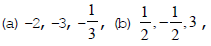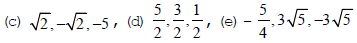25.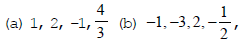(c) √2,-√2,3,-2

26. (a) 1/2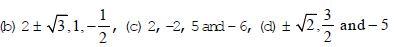27. (a) q(x) = 3x – 5, (b) f(x) = – x3 + 3x2 – 3x + 5,  (c) g(x) = x3 – 3x + 1, (d) r(x) = x + 1,

PREVIOUS YEARS BOARD (CBSE) QUESTIONS

1. x = – 1

2. 3, – 2

3. x2 – 3x – 2

4. 3

5. 2

6. 9

7. 3

8. a = 1

9. 2x2 + 3x – 9

10. 5x2 + 3x – 13

The document Assignment - Polynomials, Class 10 Mathematics Notes - Class 10 is a part of Class 10 category.
All you need of Class 10 at this link: Class 10Use Code STAYHOME200 and get INR 200 additional OFF

Track your progress, build streaks, highlight & save important lessons and more!

,

,

,

,

,

,

,

,

,

,

,

,

,

,

,

,

,

,

,

,

,

,

,

,

;